# Make a Cumulative Chart

This post will guide you how to make a cumulative sum chart in Excel. How do I create a cumulative sum chart in Excel 2013/2016. How to add a cumulative total to a line chart in Excel.

## Create a Cumulative Chart

Assuming that you have a list of data in range B1:C5 that contain sales data. And you want to create a cumulative sum chart for these data. How to achieve it. You just need to do the following steps:

#1 select one blank cell next to the Sales column, and type the name of column D header as “TOTAL”.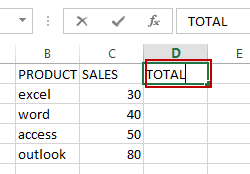#2 type the following formula based on the SUM function in the Cell D2. And press Enter key in your keyboard, and then drag the AutoFill Handle from Cell D2 to D5.

`=SUM(C\$2:C2)`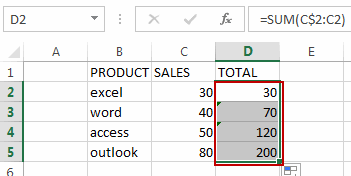#3 select all data, and then go to INSERT tab, click Insert Column Chart command under Charts group. And select one 2-D Clustered Column. And one Column Chart has been created based on the selected data.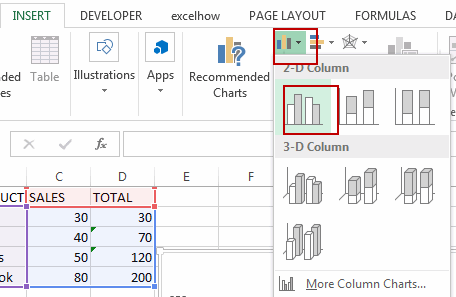#4 click Total Data series in the chart, and right click on it and select Change Series Chart Type from the popup menu list. The Change Chart Type dialog will open.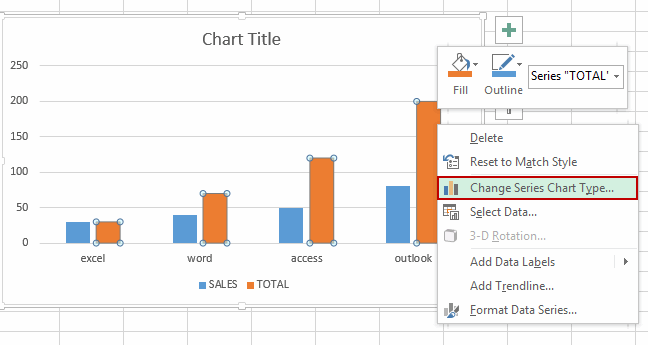#5 change the Chart type for TOTAL data series to Line, click OK button.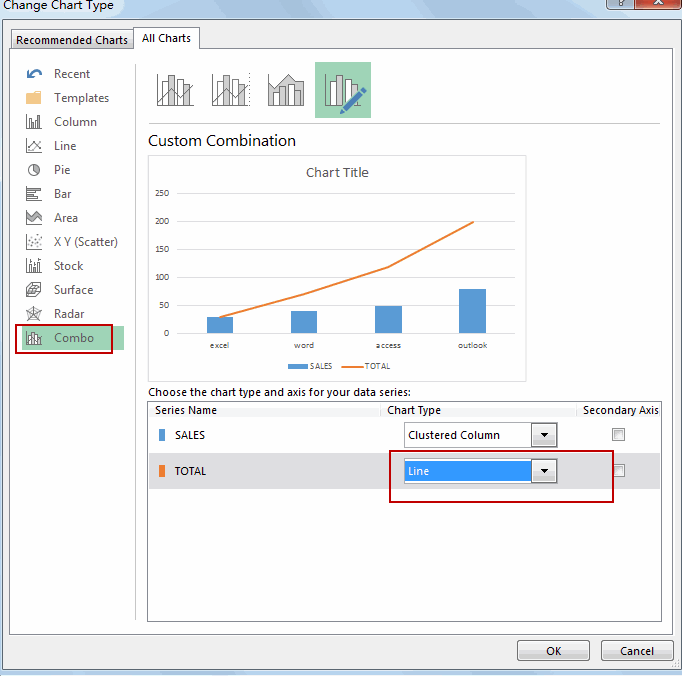#6 select the line data series, and right click on it. And select Add Data Labels, then select Add Data Labels sub menu.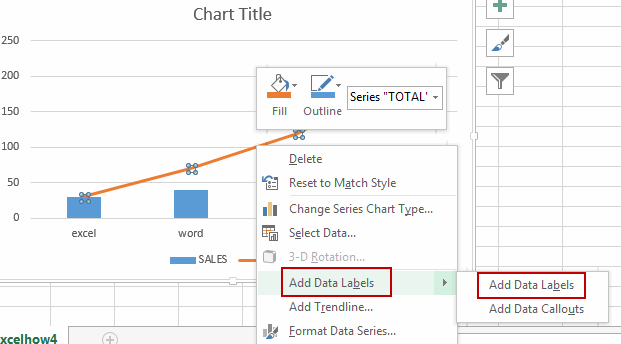#7 Let’s see the result.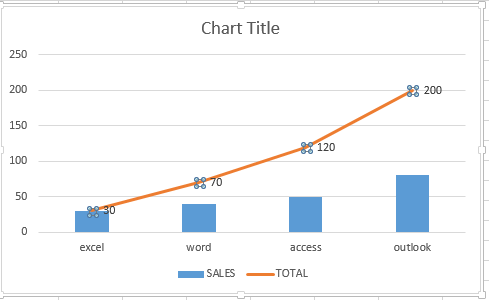### Related Functions

• Excel SUM function
The Excel SUM function will adds all numbers in a range of cells and returns the sum of these values. You can add individual values, cell references or ranges in excel.The syntax of the SUM function is as below:= SUM(number1,[number2],…)…
Related Posts
If Cell Begins with One of Three Supplied Characters

If you want to test values to see if they begin with some given specific characters like “x”, ”y”, or “z”, you can create a formula with COUNTIF and SUM functions to return results. EXAMPLE You can see “TRUE” or ...

Average per Week by Formula in Excel

We usually apply AVERAGE function or relevant functions to return average directly in Excel worksheet. But in some situations, only applying average relevant functions cannot figure out our problem. Sometimes we can create a formula with functions and mathematical operation ...

How to Sum if Contains an Asterisk

To add numbers together we need to apply SUM function. And if we want to add numbers based on some conditions, we can add criteria with the help of SUMIFS function, SUMIFS can filter data with multiple criteria effectively. If ...

How to Sum in Vertical Range

If we want to add numbers based on some conditions in Excel worksheet, we can add criteria with the help of SUMIFS function, SUMIFS can filter data with multiple criteria effectively. In this article, we will introduce you the method ...

How to Sum in Horizontal Range

To add numbers together we need to apply SUM function. But if we want to add numbers based on some conditions, we can add criteria with the help of SUMIFS function, SUMIFS can filter data with multiple criteria effectively. In ...

How to Sum with Criteria and Or Logic in Excel

To add numbers together we need to apply SUM function. And if we want to add numbers based on some conditions, we can add criteria with the help of SUMIFS function, SUMIFS can filter data with multiple criteria effectively. If ...

How To Sum the Largest N Values in Excel

Sometimes we may want to sum the largest N numbers or top N numbers in a range. In this article, we will show you the method of “SUM the Largest N Numbers” by a simple formula which consist of SUMPRODUCT ...

How to Sum for Cell Contains Formula Only in Excel

Sometimes values are created by formulas in cells. If we want to sum values which are created by formulas from a range, but some values which are hardcoded also list in the same range, how can we filter out matched ...

How to Sum the Smallest N Values in Excel

Sometimes we may want to sum the first smallest N numbers in a range in Excel. In this article, we will show you the method of “SUM the Smallest N Numbers” by a simple formula which consist of SUMPRODUCT and ...

How to Subtotal Values for Groups and Only Keep One Subtotal for A Group in Column

Sometimes we may meet the case that subtotal values for different groups and only record a subtotal value for one group in a column, for other cells in this group, keep them blank. Today we will introduce you how can ...

Sidebar